# JP6018662B2 - Counting device, physical quantity sensor, counting method and physical quantity measuring method - Google Patents

Counting device, physical quantity sensor, counting method and physical quantity measuring method Download PDF

## Info

Publication number
JP6018662B2
JP6018662B2 JP2015071575A JP2015071575A JP6018662B2 JP 6018662 B2 JP6018662 B2 JP 6018662B2 JP 2015071575 A JP2015071575 A JP 2015071575A JP 2015071575 A JP2015071575 A JP 2015071575A JP 6018662 B2 JP6018662 B2 JP 6018662B2
Authority
JP
Japan
Prior art keywords
period
counting
signal
representative value
value
Prior art date
Legal status (The legal status is an assumption and is not a legal conclusion. Google has not performed a legal analysis and makes no representation as to the accuracy of the status listed.)
Active
Application number
JP2015071575A
Other languages
Japanese (ja)
Other versions
JP2015145877A (en
Inventor

Original Assignee
アズビル株式会社
Priority date (The priority date is an assumption and is not a legal conclusion. Google has not performed a legal analysis and makes no representation as to the accuracy of the date listed.)
Filing date
Publication date
Application filed by アズビル株式会社 filed Critical アズビル株式会社
Priority to JP2015071575A priority Critical patent/JP6018662B2/en
Publication of JP2015145877A publication Critical patent/JP2015145877A/en
Application granted granted Critical
Publication of JP6018662B2 publication Critical patent/JP6018662B2/en
Application status is Active legal-status Critical
Anticipated expiration legal-status Critical

## Images

••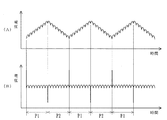•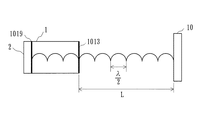•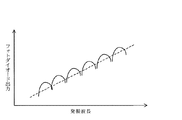•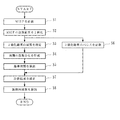•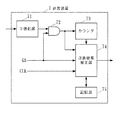•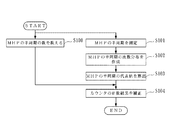•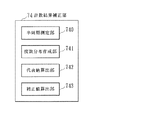•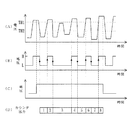•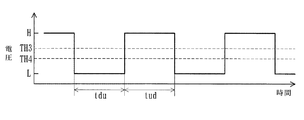•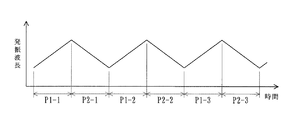•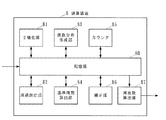•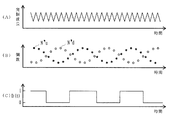•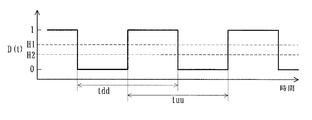•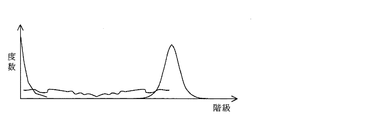•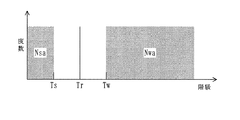••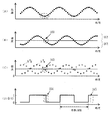•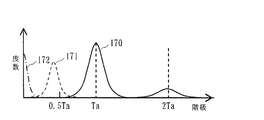••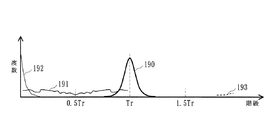•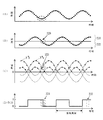•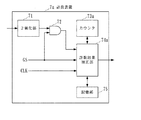••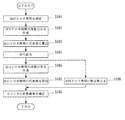•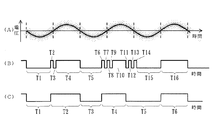•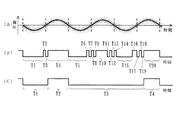•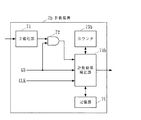•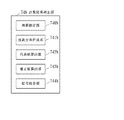•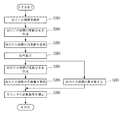•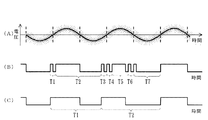•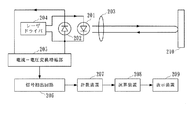•## Description

The present invention relates to a counting device that counts the number of signals, and an interference-type physical quantity sensor that measures the number of interference waveforms by using the counting device to obtain a physical quantity to be measured.

Conventionally, a wavelength modulation type laser measuring instrument using the self-coupling effect of a semiconductor laser has been proposed (see Patent Document 1). The structure of this laser measuring instrument is shown in FIG. 32 includes a semiconductor laser 201 that emits laser light to an object 210, a photodiode 202 that converts the light output of the semiconductor laser 201 into an electrical signal, and a light that is collected from the semiconductor laser 201. 210 irradiates the lens 210 and collects the return light from the object 210 and makes it incident on the semiconductor laser 201. The first oscillation period and the oscillation wavelength continuously increase in the semiconductor laser 201. Of the laser driver 204 that alternately repeats the second oscillation period that decreases to a current, a current-voltage conversion amplification unit 205 that converts and amplifies the output current of the photodiode 202 into a voltage, and a current-voltage conversion amplification unit 205 A signal extraction circuit 206 for differentiating the output voltage twice, and a mode hop pulse (hereinafter referred to as MHP) included in the output voltage of the signal extraction circuit 206 Having a counting device 207 which counts the number of which), an arithmetic unit 208 which calculates the speed of the distance and the object 210 with the object 210, and a display device 209 for displaying the calculation result of the arithmetic unit 208.

The laser driver 204 supplies a triangular wave drive current that repeatedly increases and decreases at a constant change rate with respect to time to the semiconductor laser 201 as an injection current. Accordingly, the semiconductor laser 201 alternately repeats the first oscillation period in which the oscillation wavelength continuously increases at a constant change rate and the second oscillation period in which the oscillation wavelength continuously decreases at a constant change rate. To be driven. FIG. 33 is a diagram showing the change over time of the oscillation wavelength of the semiconductor laser 201. In FIG. 33, P1 is the first oscillation period, P2 is the second oscillation period, λa is the minimum value of the oscillation wavelength in each period, λb is the maximum value of the oscillation wavelength in each period, and Tt is the period of the triangular wave.

Laser light emitted from the semiconductor laser 201 is collected by the lens 203 and enters the object 210. The light reflected by the object 210 is collected by the lens 203 and enters the semiconductor laser 201. The photodiode 202 converts the optical output of the semiconductor laser 201 into a current. The current-voltage conversion amplification unit 205 converts and amplifies the output current of the photodiode 202 into a voltage, and the signal extraction circuit 206 differentiates the output voltage of the current-voltage conversion amplification unit 205 twice. The counting device 207 counts the number of MHPs included in the output voltage of the signal extraction circuit 206 for each of the first oscillation period P1 and the second oscillation period P2. Based on the minimum oscillation wavelength λa and the maximum oscillation wavelength λb of the semiconductor laser 1, the number of MHPs in the first oscillation period P1, and the number of MHPs in the second oscillation period P2, the arithmetic unit 208 The speed of the object 210 is calculated. If the number of MHPs is measured using such a self-coupled laser measuring device technique, the vibration frequency of the object can be calculated from the number of MHPs.

In the laser measuring instrument as described above, for example, noise such as ambient light is counted as MHP, or there is MHP that cannot be counted due to missing teeth of the signal, and an error occurs in the number of MHPs counted by the counting device, There is a problem that an error occurs in the physical quantity such as the calculated distance and vibration frequency.
Therefore, the inventor measures the period of MHP during the counting period, creates a frequency distribution of the period during the counting period from the measurement result, calculates a representative value of the period of MHP from the frequency distribution, and represents the representative value from the frequency distribution. A total sum Ns of class frequencies that is less than or equal to a first predetermined number of values and a total sum Nw of class frequencies that are greater than or equal to a second predetermined number of values of the representative value are obtained, and based on these frequencies Ns and Nw By correcting the counting result of MHP, a counting device has been proposed that can eliminate the influence of missing or excessive counting during counting (see Patent Document 2).

JP 2006-31080 A JP 2009-47676 A

According to the counting device disclosed in Patent Document 2, generally good correction can be performed as long as SN (Signal to Noise ratio) does not extremely decrease.
However, in the counting device disclosed in Patent Document 2, when the signal strength is extremely strong compared to the hysteresis width in short distance measurement, the signal input to the counting device is compared with the binarization threshold value by MHP. However, chattering occurs due to high-frequency noise, and a signal with a short period or a signal with a period of about half the original period of MHP may occur frequently. In this case, since a period shorter than the original period of the MHP becomes a representative value of the distribution of the period, the MHP count result cannot be corrected correctly, and the MHP count result is, for example, several There was a problem of becoming twice as large.

The present invention has been made in order to solve the above-described problem, and is capable of correcting the counting error even when high-frequency noise is continuously generated in the signal input to the counting device. It is an object of the present invention to provide a physical quantity sensor and a physical quantity measuring method that can improve the measurement accuracy of a physical quantity by correcting the counting error of the apparatus, the counting method, and MHP.

The present invention relates to a counting device for counting a waveform of one period of the signal having a linear relationship between a specific physical quantity and the number of single signals, and having a substantially single frequency when the specific physical quantity is constant. The signal period measuring means for measuring the period of a single input signal in a certain counting period each time a signal is inputted, and the frequency of the input signal in the counting period from the measurement result of the signal period measuring means Frequency distribution creating means for creating a distribution, representative value calculating means for calculating a representative value of the period distribution of the input signal from the frequency distribution created by the frequency distribution creating means, and measurement results of the signal period measuring means, A cycle obtained by combining a cycle having a length less than 0.5 times the representative value and a cycle measured immediately thereafter is defined as a combined cycle, and a signal waveform obtained by combining the cycles is a waveform for one cycle of one signal. And after combining Signal combining means for performing until the period is equal to or greater than 0.5 times the representative value, the signal counting means for counting a period of the waveform of a single signal after processing of said signal coupling means in the counting period, the From the processing result of the signal combining means, the sum Ns of the number of periods that is less than 0.5 times the representative value and (n + 0.5) times or more (n + 1.5) times (n is 1 or more) the representative value. It obtains a total sum Nw n of the number of cycles is a natural number) of a correction value calculating means for correcting the counting result of the signal counting means on the basis of the frequencies Ns and Nw n, the frequency distribution generating means Further, the frequency distribution of the period of the input signal during the counting period is created from the processing result of the signal combining means, and the representative value calculating means is created from the processing result of the signal combining means by the frequency distribution creating means From the frequency distribution, the frequency of the input signal A representative value by calculating the representative value is updated to the latest value of the correction value calculation means corrects the counting result of the signal counting means with the latest representative values said representative value calculation means has calculated When the counting result of the signal counting means is N, the representative value is T0, and the maximum value that can be taken by the period of the single signal is T max , the corrected counting result N ′ is
It is characterized by calculating | requiring by .
Further, in one configuration example of the counting device of the present invention, the representative value is any one of a median value, a mode value, an average value, and a class value that maximizes the product of the class value and the frequency. .

The physical quantity sensor of the present invention includes a semiconductor laser that emits laser light to a measurement target, a first oscillation period in which the oscillation wavelength continuously increases monotonously, and a second oscillation period in which the oscillation wavelength continuously decreases monotonously. Oscillation wavelength modulation means for operating the semiconductor laser so that at least one of them repeatedly exists, and an interference waveform generated by a self-coupling effect between the laser light emitted from the semiconductor laser and the return light from the measurement object Detection means for detecting an electrical signal, and the interference waveform as a single signal to be counted, and a waveform of one cycle of this signal, the first oscillation period and the second oscillation period as a counting period And a calculating means for obtaining the physical quantity of the measurement object from the counting result of the counting apparatus.

In the present invention, the specific physical quantity and the number of single signals have a linear relationship, and when the specific physical quantity is constant, the waveform of one period of the signal having a substantially single frequency is counted. In the counting method, a signal cycle measuring procedure for measuring a cycle of a single input signal in a certain counting period every time a signal is inputted, and a cycle of the input signal in the counting period from a measurement result of the signal cycle measuring procedure A first frequency distribution creating procedure for creating a frequency distribution of the input signal, and a first representative value calculating procedure for calculating a representative value of the period distribution of the input signal from the frequency distribution created in the first frequency distribution creating procedure, In the measurement result of the signal cycle measurement procedure, a signal obtained by combining a cycle with a cycle obtained by combining a cycle having a length less than 0.5 times the representative value and a cycle measured immediately thereafter as a cycle after combination. Wave for one cycle of one signal The signal combining procedure that is performed until the combined period becomes 0.5 times or more of the representative value, and the frequency distribution of the period of the input signal during the counting period is created from the processing result of the signal combining procedure. A second frequency distribution creating procedure, and a second value for calculating a representative value of the distribution of the period of the input signal from the frequency distribution created by the second frequency distribution creating procedure and updating the representative value to the latest value. From the representative value calculation procedure, the signal counting procedure for counting the waveform of one cycle of the single signal after the signal combining procedure in the counting period, and the processing result of the signal combining procedure, the latest representative value The sum Ns of the number of cycles that is less than 0.5 times and the sum of the number of cycles that is (n + 0.5) times or more and (n + 1.5) times (n is a natural number of 1 or more) times the latest representative value. seeking and nw n, before on the basis of the frequencies Ns and Nw n And a correction value calculation procedure for correcting the counting result of the signal counting procedure, the correction value calculation procedure, the counting result of the signal counting procedure N, the representative value T0, the period of the single signal may take When the maximum value is T max , the corrected count result N ′ is
It is characterized by calculating | requiring by .
Moreover, in one configuration example of the counting method of the present invention, the representative value is any one of a median value, a mode value, an average value, and a class value that maximizes the product of the class value and the frequency. .

Further, the physical quantity measuring method of the present invention is a semiconductor in which at least one of the first oscillation period in which the oscillation wavelength continuously increases monotonically and the second oscillation period in which the oscillation wavelength continuously decreases monotonously exists. An oscillation procedure for operating a laser, a detection procedure for detecting an electrical signal including an interference waveform caused by a self-coupling effect between laser light emitted from the semiconductor laser and return light from the measurement object, and an object for counting the interference waveform A signal extraction procedure for counting the first oscillation period and the second oscillation period as a counting period, and a counting result of the signal extraction procedure. and a calculation procedure for obtaining the physical quantity of the measurement object, wherein the signal extraction procedure is characterized in the use of pre-Symbol counting method.

According to the present invention, the period of the input signal during the counting period is measured, and the frequency distribution of the period of the input signal during the counting period is created from the measurement result, and the representative value of the distribution of the period of the input signal from the frequency distribution For the measurement result of the cycle, the cycle that is less than 0.5 times the representative value and the cycle measured immediately after that is taken as the combined cycle, and the signal waveform that combines the cycles is The waveform of one cycle of one signal is made until the combined cycle becomes 0.5 times or more of the representative value, and the frequency of the input signal during the counting period is determined from the processing result of the signal combining means. Create the distribution again, calculate the representative value of the period of the input signal from this frequency distribution, update the representative value to the latest value, count the signal after processing of the signal combining means in the counting period, and process the signal combining means From the results, the circumference is less than 0.5 times the latest representative value. And the sum Ns of the number of seeking the latest representative values of (n + 0.5) times as long or more (n + 1.5) of the number of cycles is less than doubled sum Nw n, signals on the basis of the frequencies Ns and Nw n By correcting the counting result of the counting means, the counting error can be corrected with high accuracy even when high-frequency noise is continuously generated in the signal input to the counting device.

Further, in the present invention, even when high-frequency noise is continuously generated in the signal input to the counting device by using the counting device capable of correcting the counting error with high accuracy, the signal input to the counting device. The physical quantity to be measured can be measured with high accuracy.

It is a block diagram which shows the structure of the vibration frequency measuring device which concerns on the 1st reference example of this invention. It is a wave form diagram which shows typically the output voltage waveform of the current-voltage conversion amplification part in the 1st reference example of the present invention, and the output voltage waveform of a filter part. It is a figure for demonstrating a mode hop pulse. It is a figure which shows the relationship between the oscillation wavelength of a semiconductor laser, and the output waveform of a photodiode. It is a flowchart which shows operation | movement of the counting device and arithmetic unit in the 1st reference example of this invention. It is a block diagram which shows one example of a structure of the counting device in the 1st reference example of this invention. It is a flowchart which shows operation | movement of the counting apparatus in the 1st reference example of this invention. It is a block diagram which shows an example of a structure of the count result correction | amendment part of the counting device in the 1st reference example of this invention. It is a figure for demonstrating operation | movement of the counter of the counting device in the 1st reference example of this invention. It is a figure for demonstrating operation | movement of the half cycle measuring part of the counting device in the 1st reference example of this invention. It is a figure for demonstrating the relationship between the period which the representative value calculation part of the counting device in the 1st reference example of this invention calculates a representative value, and the count period of correction | amendment object. It is a block diagram which shows one example of a structure of the arithmetic unit in the 1st reference example of this invention. It is a figure for demonstrating operation | movement of the binarization part of the arithmetic unit in the 1st reference example of this invention. It is a figure for demonstrating operation | movement of the period measurement part of the arithmetic unit in the 1st reference example of this invention. It is a figure which shows one example of the frequency distribution of the period of the binarization output which binarized the count result of the counting device in the 1st reference example of this invention. It is a figure which represents typically the frequency used for correction | amendment of the count result of the counter of the arithmetic unit in the 1st reference example of this invention. It is a figure for demonstrating the correction principle of the count result of the counter of the arithmetic unit in the 1st reference example of this invention. It is a figure for demonstrating the problem of the conventional counting device. It is a figure which shows one example of the frequency distribution of the period of a mode hop pulse when the high frequency noise mixes in the signal input into a counting device. It is a figure which shows one example of the frequency distribution of the half period of a mode hop pulse. It is a figure which shows the frequency distribution of the period produced according to the binarization output of FIG. The figure for demonstrating the signal obtained with the vibration frequency measuring device which concerns on the 1st reference example of this invention, when the ratio of the maximum speed of vibration of an object and the distance of an object is larger than the wavelength change rate of a semiconductor laser. It is. It is a block diagram which shows one example of a structure of the counting device in the 2nd reference example of this invention. It is a block diagram which shows one example of a structure of the count result correction | amendment part of the counting apparatus in the 2nd reference example of this invention. It is a flowchart which shows operation | movement of the counting apparatus in the 2nd reference example of this invention. It is a figure for demonstrating operation | movement of the signal coupling | bond part of the counting device in the 2nd reference example of this invention. It is a figure for demonstrating operation | movement of the signal coupling | bond part of the counting device in the 3rd reference example of this invention. It is a block diagram which shows an example of a structure of the counting device in embodiment of this invention. It is a block diagram which shows one example of a structure of the count result correction | amendment part of the counting device in embodiment of this invention. It is a flowchart which shows operation | movement of the counting device in embodiment of this invention. It is a figure for demonstrating operation | movement of the signal coupling | bond part of the counting device in embodiment of this invention. It is a block diagram which shows the structure of the conventional laser measuring device. It is a figure which shows one example of the time change of the oscillation wavelength of a semiconductor laser in the laser measuring device of FIG.

[First Reference Example]
Hereinafter, reference examples of the present invention will be described with reference to the drawings. FIG. 1 is a block diagram showing a configuration of a vibration frequency measuring apparatus according to a first reference example of the present invention.
The vibration frequency measuring apparatus of FIG. 1 collects light from a semiconductor laser 1 that emits laser light to an object 10 to be measured, a photodiode 2 that converts the light output of the semiconductor laser 1 into an electrical signal, and light from the semiconductor laser 1. A lens 3 that radiates and emits light, collects return light from the object 10 and makes it incident on the semiconductor laser 1, a laser driver 4 that serves as an oscillation wavelength modulation unit that drives the semiconductor laser 1, and an output of the photodiode 2 A current-voltage conversion amplification unit 5 that converts current into voltage and amplifies it; a filter unit 6 that removes a carrier wave from the output voltage of the current-voltage conversion amplification unit 5; and a self-coupled signal included in the output voltage of the filter unit 6 A counting device 7 that counts the number of mode hop pulses (MHP), a computing device 8 that determines the vibration frequency of the object 10 based on the counting result of the counting device 7, and a computing device 8 And a display device 9 for displaying the results.

The photodiode 2 and the current-voltage conversion amplification unit 5 constitute detection means. Hereinafter, for ease of explanation, it is assumed that a semiconductor laser 1 of a type that does not have a mode hopping phenomenon (VCSEL type, DFB laser type) is used.

The laser driver 4 supplies a triangular wave drive current that repeatedly increases and decreases at a constant change rate with respect to time to the semiconductor laser 1 as an injection current. As a result, the semiconductor laser 1 has a first oscillation period P1 in which the oscillation wavelength continuously increases at a constant change rate in proportion to the magnitude of the injection current, and the oscillation wavelength continuously decreases at a constant change rate. It is driven to alternately repeat the second oscillation period P2. The time variation of the oscillation wavelength of the semiconductor laser 1 at this time is as shown in FIG. In this reference example, the maximum value λb of the oscillation wavelength and the minimum value λa of the oscillation wavelength are always constant, and the difference λb−λa is also always constant.

Laser light emitted from the semiconductor laser 1 is collected by the lens 3 and enters the object 10. The light reflected by the object 10 is collected by the lens 3 and enters the semiconductor laser 1. However, condensing by the lens 3 is not essential. The photodiode 2 is disposed in the semiconductor laser 1 or in the vicinity thereof, and converts the optical output of the semiconductor laser 1 into a current. The current-voltage conversion amplification unit 5 converts the output current of the photodiode 2 into a voltage and amplifies it.

The filter unit 6 has a function of extracting a superimposed signal from the modulated wave. FIG. 2A is a diagram schematically showing the output voltage waveform of the current-voltage conversion amplification unit 5, and FIG. 2B is a diagram schematically showing the output voltage waveform of the filter unit 6. These figures are obtained by removing the oscillation waveform (carrier wave) of the semiconductor laser 1 of FIG. 2 from the waveform (modulated wave) of FIG. 2A corresponding to the output of the photodiode 2, and the MHP of FIG. A process of extracting a waveform (interference waveform) is shown.

Here, the MHP that is a self-coupled signal will be described. As shown in FIG. 3, when the distance from the mirror layer 1013 to the object 10 is L and the oscillation wavelength of the laser is λ, the return light from the object 10 and the optical resonance of the semiconductor laser 1 are satisfied when the following resonance conditions are satisfied. The laser light in the chamber strengthens and the laser output increases slightly.
L = qλ / 2 (1)
In Formula (1), q is an integer. This phenomenon can be sufficiently observed even if the scattered light from the object 10 is extremely weak, because the apparent reflectance in the resonator of the semiconductor laser 1 increases, causing an amplification effect.

FIG. 4 is a diagram showing the relationship between the oscillation wavelength and the output waveform of the photodiode 2 when the oscillation wavelength of the semiconductor laser 1 is changed at a certain rate. When L = qλ / 2 shown in Expression (1) is satisfied, the phase difference between the return light and the laser light in the optical resonator becomes 0 ° (the same phase), and the return light and the optical resonator The laser beam is the most intense, and when L = qλ / 2 + λ / 4, the phase difference is 180 ° (reverse phase), and the return light and the laser beam in the optical resonator are most weakened. Therefore, when the oscillation wavelength of the semiconductor laser 1 is changed, a place where the laser output becomes stronger and a place where the laser output becomes weaker appear alternately. When the laser output at this time is detected by the photodiode 2, as shown in FIG. A stepped waveform with a constant period can be obtained. Such a waveform is generally called an interference fringe. Each stepped waveform, that is, each interference fringe is MHP. When the oscillation wavelength of the semiconductor laser 1 is changed for a certain period of time, the number of MHPs changes in proportion to the measurement distance.

Next, operations of the counting device 7 and the arithmetic device 8 will be described. FIG. 5 is a flowchart showing the operations of the counting device 7 and the arithmetic device 8.
The counting device 7 counts the number of MHPs included in the output voltage of the filter unit 6 for each of the first oscillation period P1 and the second oscillation period P2 (step S1 in FIG. 5). FIG. 6 is a block diagram showing an example of the configuration of the counting device 7. The counting device 7 includes a binarization unit 71, an AND operation unit (AND) 72, a counter 73, a counting result correction unit 74, and a storage unit 75. The current-voltage conversion amplification unit 5, the filter unit 6, the binarization unit 71 of the counting device 7, the AND 72, and the counter 73 constitute a signal counting unit.

FIG. 7 is a flowchart showing the operation of the counting device 7, and FIG. 8 is a block diagram showing an example of the configuration of the counting result correction unit 74. The counting result correction unit 74 includes a half-cycle measurement unit 740, a frequency distribution creation unit 741, a representative value calculation unit 742, and a correction value calculation unit 743.

9A to 9D are diagrams for explaining the operation of the counter 73, and FIG. 9A schematically shows the waveform of the output voltage of the filter unit 6, that is, the waveform of MHP. 9B is a diagram showing the output of the binarization unit 71 corresponding to FIG. 9A, FIG. 9C is a diagram showing the gate signal GS input to the counting device 7, and FIG. () Is a diagram showing a counting result of the counter 73 corresponding to FIG.

First, the binarization unit 71 of the counting device 7 determines whether the output voltage of the filter unit 6 shown in FIG. 9A is a high level (H) or a low level (L), and the binarization unit 71 of FIG. Such a determination result is output. At this time, the binarization unit 71 determines that the output voltage of the filter unit 6 is high level when the output voltage of the filter unit 6 is higher than or equal to the threshold value TH1, and the output voltage of the filter unit 6 decreases and the threshold value TH2 By determining that the level is low when (TH2 <TH1) or less, the output of the filter unit 6 is binarized.

The AND 72 outputs a logical product operation result of the output of the binarizing unit 71 and the gate signal GS as shown in FIG. 9C, and the counter 73 counts the rise and fall of the output of the AND 72 (FIG. 9 (D)). Here, the gate signal GS is a signal that rises at the beginning of the counting period (the first oscillation period P1 or the second oscillation period P2 in this reference example) and falls at the end of the counting period. Therefore, the counter 73 counts the number of rising edges and the number of falling edges (that is, the number of half cycles of MHP) of the output of the AND 72 during the counting period (step S100 in FIG. 7).

FIG. 10 is a diagram for explaining the operation of the half cycle measuring unit 740 of the counting result correcting unit 74. The half cycle measurement unit 740 measures the half cycle of the MHP during the counting period (step S101 in FIG. 7). That is, the half-cycle measuring unit 740 detects the rising edge of the output of the AND72 by comparing the output of the AND72 during the counting period with the threshold value TH3, and compares the output of the AND72 with the threshold value TH4. , The falling edge of the output of AND72 is detected. The half-cycle measuring unit 740 measures a time tud from the rising edge of the output of the AND 72 to the next falling edge, and measures a time tdu from the falling edge of the output of the AND 72 to the next rising edge, thereby counting time period. Measure the half cycle of the output of AND 72 in the middle (ie, half cycle of MHP). Thus, the half cycle of MHP is time tud or tdu. The half-cycle measuring unit 740 performs the above measurement every time when either the rising edge or falling edge of the output of the AND 72 is detected.
The storage unit 75 stores the count result of the counter 73 and the measurement result of the half cycle measurement unit 740.

After the gate signal GS falls and the counting period ends, the frequency distribution creating unit 741 of the counting result correcting unit 74 determines the half of the MHP during the counting period from the measurement result of the half cycle measuring unit 740 stored in the storage unit 75. A frequency distribution of periods is created (step S102 in FIG. 7).

Subsequently, the representative value calculation unit 742 of the counting result correction unit 74 calculates the representative value T0 of the MHP half cycle from the frequency distribution created by the frequency distribution creation unit 741 (step S103 in FIG. 7). Here, the mode value, median value, or average value of the half-cycle of MHP may be set as the representative value T0. Further, the representative value calculation unit 742 may set the class value that maximizes the product of the class value and the frequency as the representative value T0. Table 1 shows a numerical example of the frequency distribution and the product of the class value and the frequency in this numerical example.

In the example of Table 1, the mode value (class value) having the maximum frequency is 1. On the other hand, the class value that maximizes the product of the class value and the frequency is 6, which is different from the mode value. The reason why the class value that maximizes the product of the class value and the frequency is set as the representative value T0 will be described later. The representative value T0 calculated by the representative value calculation unit 742 is stored in the storage unit 75. The representative value calculation unit 742 performs such calculation of the representative value T0 every time a frequency distribution is created by the frequency distribution creation unit 741.

From the measurement result of the half cycle measurement unit 740, the correction value calculation unit 743 of the count result correction unit 74 calculates the total number Ns of half cycles that is less than 0.5 times the representative value T0 and 2n times or more of the representative value T0. (2n + 2) less times (n is a natural number not exceeding 1 or n max) determined the sum Nw n of the number of half cycles is to correct the counting result of the counter 73 as follows (Fig. 7 step S104).

In Equation (2), N is the number of half-cycles of MHP, which is the counting result of the counter 73, N ′ is the number of MHPs obtained after correction, and T max is the maximum value that can be taken by the half-cycle of MHP. The correction principle of the counting result of the counter 73 will be described later.
The counting device 7 performs the above processing for each of the first oscillation period P1 and the second oscillation period P2.

Note that the representative value T0 used by the correction value calculation unit 743 is a value calculated from the measurement result of the half cycle measurement unit 740 in the counting period one period before the carrier wave (triangular wave) before the counting period to be corrected. Alternatively, a value calculated from the measurement result of the half cycle measuring unit 740 in the counting period to be corrected may be used. FIG. 11 is a diagram illustrating a temporal change in the oscillation wavelength of the semiconductor laser 1, and is a diagram for explaining a relationship between a period during which the representative value calculation unit 742 calculates the representative value T0 and a counting period to be corrected.

In the case of using the representative value T0 calculated from the measurement result one cycle before the correction target counting period, the correction value calculation unit 743 is calculated, for example, in the first oscillation period P1-1 shown in FIG. The count result of the first oscillation period P1-2 is corrected using the representative value T0, and the count of the second oscillation period P2-2 is corrected using the representative value T0 calculated in the second oscillation period P2-1. The result will be corrected. Further, when using the representative value T0 calculated from the measurement result of the counting period to be corrected, the correction value calculation unit 743 uses, for example, the representative value T0 calculated in the first oscillation period P1-1 shown in FIG. To correct the counting result of the first oscillation period P1-1 and to correct the counting result of the second oscillation period P2-1 using the representative value T0 calculated in the second oscillation period P2-1. Become.

However, even when using the representative value T0 calculated from the measurement result of one cycle of the carrier wave before the counting period of the correction target, the initial value of the representative value T0 does not exist in the first process, so the correction target The representative value T0 is obtained from the measurement result of the half cycle measurement unit 740 in the counting period, and the counting result is corrected.

Next, the computing device 8 calculates the vibration frequency of the object 10 based on the number of MHPs counted by the counting device 7. FIG. 12 is a block diagram showing an example of the configuration of the arithmetic unit 8. The arithmetic device 8 includes a storage unit 80 that stores the counting result of the counting device 7, a binarizing unit 81 that binarizes the counting result of the counting device 7, and the binarization output from the binarizing unit 81. A cycle measuring unit 82 that measures the cycle of the output, a frequency distribution creating unit 83 that creates a frequency distribution of the cycle of the binarized output, and a reference for calculating a reference cycle that is a representative value of the cycle distribution of the binarized output Period calculation unit 84, counter 85 serving as a binarized output counting means for counting the number of binarized output pulses, correction unit 86 for correcting the count result of counter 85, and object based on the corrected count result A frequency calculation unit 87 that calculates ten vibration frequencies.

The counting result of the counting device 7 is stored in the storage unit 80 of the arithmetic device 8. The binarizing unit 81 of the arithmetic device 8 binarizes the counting result of the counting device 7 stored in the storage unit 80 (step S2 in FIG. 5). 13A and 13B are diagrams for explaining the operation of the binarization unit 81. FIG. 13A is a diagram showing a change with time of the oscillation wavelength of the semiconductor laser 1, and FIG. 13B is a counting result of the counting device 7. FIG. 13C is a diagram showing the output D (t) of the binarization unit 81. FIG. In FIG. 13B, N'u is the counting result of the first oscillation period P1, and N'd is the counting result of the second oscillation period P2.

The binarization unit 81 compares the count results N′u and N′d in two oscillation periods P1 and P2 that are temporally adjacent to each other, and binarizes these count results. Specifically, the binarizing unit 81 executes the following expression.
If N′u (t) ≧ N′d (t−1) then D (t) = 1 (3)
If N′u (t) <N′d (t−1) then D (t) = 0 (4)
If N′d (t) ≦ N′u (t−1) then D (t) = 1 (5)
If N′d (t)> N′u (t−1) then D (t) = 0 (6)

In Expressions (3) to (6), (t) represents the number of MHPs measured at the current time t, and (t−1) represents the MHP measured one time before the current time t. It represents a number. In Expressions (3) and (4), the count result at the current time t is the count result N′u in the first oscillation period P1, and the previous count result is the count result N ′ in the second oscillation period P2. This is the case of d. In this case, if the counting result N′u (t) at the current time t is greater than or equal to the previous counting result N′d (t−1), the binarizing unit 81 outputs the output D (t at the current time t. ) Is set to “1” (high level) and the count result N′u (t) at the current time t is smaller than the count result N′d (t−1) of the previous time, the output D ( t) is set to “0” (low level).

In Expressions (5) and (6), the counting result at the current time t is the counting result N′d of the second oscillation period P2, and the previous counting result is the counting result N ′ of the first oscillation period P1. This is the case for u. In this case, if the counting result N′d (t) at the current time t is equal to or less than the previous counting result N′u (t−1), the binarization unit 81 outputs the output D (t ) Is “1”, and the count result N′d (t) at the current time t is larger than the count result N′u (t−1) one time before, the output D (t) at the current time t is “ 0 ”.

Thus, the counting result of the counting device 7 is binarized. The output D (t) of the binarization unit 81 is stored in the storage unit 80. The binarization unit 81 performs the binarization process as described above at every time (every oscillation period) when the counting device 7 measures the number of MHPs.

Binarizing the counting result of the counting device 7 means determining the direction of displacement of the object 10. That is, when the count result N′u when the oscillation wavelength of the semiconductor laser 1 is increasing is equal to or greater than the count result N′d when the oscillation wavelength is decreasing (D (t) = 1), the object 10 Is the direction of approaching the semiconductor laser 1, and when the counting result N′u is smaller than the counting result N′d (D (t) = 0), the moving direction of the object 10 is a direction away from the semiconductor laser 1. is there. Therefore, basically, if the cycle of the binarized output shown in FIG. 13C can be obtained, the vibration frequency of the object 10 can be calculated.

The period measuring unit 82 measures the period of the binarized output D (t) stored in the storage unit 80 (step S3 in FIG. 5). FIG. 14 is a diagram for explaining the operation of the period measurement unit 82. In FIG. 14, H1 is a threshold value for detecting the rising edge of the binarized output D (t), and H2 is a threshold value for detecting the falling edge of the binarized output D (t).

The period measuring unit 82 detects the rising of the binarized output D (t) by comparing the binarized output D (t) stored in the storage unit 80 with the threshold value H1, and binarized output The period of the binarized output D (t) is measured by measuring the time tu from the rise of D (t) to the next rise. The period measuring unit 82 performs such measurement every time a rising edge occurs in the binarized output D (t).

Alternatively, the period measuring unit 82 detects the falling edge of the binarized output D (t) by comparing the binarized output D (t) stored in the storage unit 80 with the threshold value H2. The period of the binarized output D (t) may be measured by measuring the time tdd from the trailing edge of the digitized output D (t) to the next trailing edge. The period measuring unit 82 performs such measurement every time a falling edge occurs in the binarized output D (t).

The measurement result of the period measurement unit 82 is stored in the storage unit 80. Next, the frequency distribution creating unit 83 creates a frequency distribution of periods in a certain time T (T> Tt, for example, 100 × Tt, that is, a time corresponding to 100 triangular waves) from the measurement result of the period measuring unit 82. (FIG. 5, step S4). FIG. 15 is a diagram showing an example of the frequency distribution. The frequency distribution created by the frequency distribution creating unit 83 is stored in the storage unit 80. The frequency distribution creating unit 83 creates such a frequency distribution every T hours.

Subsequently, the reference cycle calculation unit 84 calculates a reference cycle Tr that is a representative value of the cycle of the binarized output D (t) from the frequency distribution created by the frequency distribution creation unit 83 (step S5 in FIG. 5). In general, the representative value of the period is the mode value or the median value, but in this reference example, the mode value or the median value is not suitable as the representative value of the period. Therefore, the reference period calculation unit 84 sets the class value that maximizes the product of the class value and the frequency as the reference period Tr. The reason why the class value that maximizes the product of the class value and the frequency is used as the reference period Tr will be described later. The calculated value of the reference period Tr is stored in the storage unit 80. The reference period calculation unit 84 performs such calculation of the reference period Tr every time the frequency distribution is created by the frequency distribution creation unit 83.

On the other hand, the counter 85 operates in parallel with the period measuring unit 82 and the frequency distribution creating unit 83, and binarized output in a period of the same fixed time T as the period for which the frequency distribution creating unit 83 is a target of frequency distribution creating. The number Na of rising edges of D (t) Na (that is, the number of “1” pulses of the binarized output D (t)) is counted (step S6 in FIG. 5). The counting result Na of the counter 85 is stored in the storage unit 80. The counter 85 counts such binarized output D (t) every T time.

From the frequency distribution created by the frequency distribution creating unit 83, the correcting unit 86 calculates the sum Nsa of the class frequencies that are 0.5 times or less of the reference period Tr and the frequency of the class that is 1.5 times or more of the reference period Tr. And the count result Na of the counter 85 is corrected as follows (step S7 in FIG. 5).
Na ′ = Na−Nsa + Nwa (7)
In equation (7), Na ′ is the corrected count result. The corrected count result Na ′ is stored in the storage unit 80. The correction unit 86 performs such correction every T time.

FIG. 16 is a diagram schematically showing the total frequency Nsa and Nwa. In FIG. 16, Ts is a class value 0.5 times the reference period Tr, and Tw is a class value 1.5 times the reference period Tr. It goes without saying that the class in FIG. 16 is a representative value of the period. In FIG. 16, the frequency distribution between the reference periods Tr and Ts and between the reference periods Tr and Tw is omitted in order to simplify the description.

FIG. 17 is a diagram for explaining the correction principle of the counting result of the counter 85. FIG. 17 (A) shows the binarized output D (t), and FIG. 17 (B) shows FIG. 17 (A). It is a figure which shows the count result of the corresponding counter 85. FIG.
Originally, the cycle of the binarized output D (t) varies depending on the vibration frequency of the object 10, but if the vibration frequency of the object 10 is unchanged, the pulse of the binarized output D (t) appears in the same cycle. However, due to noise, the MHP waveform may be missing or a waveform that should not be counted as a signal. As a result, the waveform of the binarized output D (t) should not be counted as a missing or signal. A waveform is generated, and an error occurs in the result of counting the pulses of the binarized output D (t).

When signal loss occurs, the cycle Tw of the binarized output D (t) at the location where the loss occurs is approximately twice the original cycle. That is, when the period of the binarized output D (t) is approximately twice or more than the reference period Tr, it can be determined that the signal is missing. Therefore, the sum of the frequencies Nwa of the class equal to or higher than the period Tw is regarded as the number of missing signals, and the missing signal can be corrected by adding this Nwa to the counting result Na of the counter 85.

Further, the cycle Ts of the binarized output D (t) at the portion where the original signal is divided by spike noise or the like is shorter than 0.5 times the signal shorter than the original cycle and 0.5 times. Becomes two of the long signals. That is, when the period of the binarized output D (t) is approximately 0.5 times or less of the reference period Tr, it can be determined that the signals are excessively counted. Therefore, it is possible to correct the noise that is erroneously counted by regarding the total sum Nsa of the frequencies of the classes equal to or less than the period Ts as the number of times the signal is excessively counted and subtracting this Nsa from the counting result Na of the counter 85. The above is the correction principle of the counting result shown in Expression (7).

Based on the corrected count result Na ′ calculated by the correction unit 86, the frequency calculation unit 87 calculates the vibration frequency fsig of the object 10 according to the following equation (step S8 in FIG. 5).
fsig = Na ′ / T (8)
The display device 9 displays the value of the vibration frequency fsig calculated by the arithmetic device 8.

Here, the correction principle of the counting result of the counter 73 of the counting device 7 will be described. The basic principle of the correction of the counting result shown in Expression (2) is the same as the correction principle of the counting result disclosed in Patent Document 2 and the correction principle of the counting result shown in Expression (7). However, according to the correction principle disclosed in Patent Document 2, if a burst noise having a frequency higher than that of MHP is mixed in a signal input to the counting device, the counting result of the counter 73 may not be corrected correctly. In particular, the number of samplings in vibration frequency measurement may take only about several times the vibration frequency, and a slight counting error may become a large frequency error. The conventional problem will be described below by taking vibration frequency measurement as an example.

18 (A) to 18 (D) are diagrams for explaining the problems of the conventional counting device disclosed in Patent Document 2, and FIG. 18 (A) shows the time change of the distance to the object 10. FIG. 18 (B) is a diagram showing the time change of the speed of the object 10, FIG. 18 (C) is a diagram showing the time change of the counting result of the counting device, and FIG. 18 (D) is the counting result of the counting device. It is a figure which shows the binarized binarization output D (t). In FIG. 18B, 160 indicates a portion where the velocity is low, 161 indicates that the moving direction of the object 10 is a direction approaching the semiconductor laser 1, and 162 indicates that the moving direction of the object 10 is far from the semiconductor laser 1. Indicates that the direction. 18A to 18D show a case where the ratio of the maximum speed of vibration of the object 10 and the distance to the object 10 is smaller than the wavelength change rate of the semiconductor laser 1 in this reference example. However, since the signal waveform is the same in the conventional counting device, the problems of the conventional counting device will be described with reference to FIGS. 18 (A) to 18 (D).

As shown in FIG. 18C, when a noise having a frequency higher than that of MHP is applied at a portion 163 where the speed of the object 10 is small, the magnitude relationship between the count results N′u and N′d may be reversed from the original relationship. is there. As a result, as shown in FIG. 18D, the sign of the binarized output D (t) becomes a value opposite to the original value at the location 164 where the sign of the binarized output D (t) switches. There is.

When binarizing MHP with a certain threshold value, the sign is likely to be inverted due to high-frequency noise where MHP takes a value close to the threshold value. Since the frequency distribution of the MHP period exists every two periods, as shown in FIG. 19, in addition to the distribution 170 having the maximum value of the frequency in the original period Ta of the MHP, the frequency distribution is about half the period Ta. A distribution 171 having a local maximum value and a short period 172 of noise appear. Then, due to the mixed high frequency noise, the maximum values of these frequencies tend to shift to a shorter time. Furthermore, high frequency noise may be mixed continuously. In the conventional counting device disclosed in Patent Document 2, if such high-frequency continuous noise is mixed, the MHP counting result cannot be corrected sufficiently.

Therefore, in this reference example, the count result is corrected using the representative value T0 of the half cycle instead of the representative value Ta of the MHP cycle. An example of the frequency distribution of a half cycle of MHP is shown in FIG. As is clear from FIG. 20, when the frequency distribution of the half cycle of MHP is obtained, the frequency maximum is near 0.5T0 even when high-frequency noise is mixed in the signal input to the counting device 7. The value no longer appears. That is, since the local maximum value near the threshold value for obtaining the sum Ns of the numbers of half cycles that is less than 0.5 times the representative value T0 has disappeared, the above-described Ns can be correctly obtained and corrected. The error can be suppressed. The above is the correction principle of the counting result shown in Expression (2). The reason why the right side of Expression (2) is halved is to convert the number of half-cycles of MHP into the number of MHPs.

As described above, in this reference example, the counter 73 counts the number of MHP half cycles during the counting period, and measures the MHP half cycle during the counting period. From this measurement result, the MHP half cycle during the counting period is measured. And a representative value T0 of a half period of MHP is calculated from the frequency distribution, and the total number Ns of half periods that is less than 0.5 times the representative value T0 and 2n times or more of the representative value T0 ( 2n + 2) of the number of half cycles is less than doubled search of the sum Nw n, by correcting the counting result of the counter 73 based on the frequencies Ns and Nw n, than MHP to a signal input to the counting device Even when high-frequency noise is continuously generated, the counting error of the MHP can be corrected with high accuracy, so that the measurement accuracy of the vibration frequency of the object 10 can be improved.

Furthermore, in this reference example, the count results of the first and second oscillation periods P1 and P2 that are temporally adjacent to each other are compared to binarize the MHP count result, and the binarized output D (t) A cycle frequency distribution at a fixed time T is measured to calculate a reference cycle Tr that is a representative value of the cycle distribution of the binarized output D (t) from the cycle frequency distribution. In the period, the number of pulses of the binarized output D (t) is counted, and from the frequency distribution, the total frequency Nsa of the class that is 0.5 times or less of the reference period Tr and 1.5 times or more of the reference period Tr. By calculating the sum Nwa of the frequencies of the class and correcting the counting result of the pulses of the binarized output D (t) based on these frequencies Nsa and Nwa, the counting error of the binarized output D (t) is reduced. Since it can be corrected, the measurement accuracy of the vibration frequency of the object 10 is improved. Rukoto can.

Next, the reason why the reference period calculation unit 84 sets the class value that maximizes the product of the class value and the frequency as the reference period Tr will be described.
In a self-coupled laser measuring device using wavelength modulation (triangular wave modulation in this reference example), the number of MHPs in each counting period is the number of MHPs proportional to the distance to the object 10 and the number of objects 10 in the counting period. It is the sum or difference with the number of MHPs proportional to the displacement (velocity). Depending on the magnitude relationship between the ratio of the maximum speed of vibration of the object 10 and the distance between the object 10 and the wavelength change rate of the semiconductor laser 1, the status of signals obtained by the measuring apparatus can be divided into the following two types.

First, a case where the ratio of the maximum speed of vibration of the object 10 and the distance between the object 10 is smaller than the wavelength change rate of the semiconductor laser 1 will be described with reference to FIGS. 18 (A) to 18 (D). When the ratio of the maximum speed of vibration of the object 10 and the distance to the object 10 is smaller than the wavelength change rate of the semiconductor laser 1, the number of MHPs proportional to the distance to the object 10 is the displacement of the object 10 during the counting period ( Since the number of MHPs proportional to the speed is always larger, the difference between the counting result N′u when the oscillation wavelength of the semiconductor laser 1 is increasing and the counting result N′d when the oscillation wavelength is decreasing. Is always proportional to the displacement of the object 10 in two counting periods (in this reference example, the oscillation periods P1 and P2). In this case, when N′u−N′d is plotted in time series, the vibration speed with the approaching direction to the semiconductor laser 1 being positive is shown. Therefore, the sign of N′u−N′d indicates the movement direction of the object 10, and the displacement of the object 10 can be binarized by this sign.

At this time, the frequency distribution of the period created by the frequency distribution creating unit 83 is as shown in FIG.
As shown in FIG. 18C, when white noise due to, for example, ambient light is added at a location 163 where the speed of the object 10 is low, a binary signal is output at a location 164 where the sign of the binarized output D (t) changes. The sign of the digitized output D (t) may be a value opposite to the original value. Further, for example, when spike noise caused by disturbance light or the like is added, the sign of the binarized output D (t) is locally inverted at a location 165 as shown in FIG.

As a result, as shown in FIG. 21, the frequency distribution of the period created by the frequency distribution creating unit 83 includes a normal distribution 190 centered on the reference period Tr, a frequency 191 due to sign inversion caused by spike noise, It becomes the sum with the frequency 192 due to the sign inversion caused by noise. Further, the frequency 193 of signal loss when binarization is performed often does not occur unless low-frequency noise having a large speed is mixed.

Next, a case where the ratio of the maximum speed of vibration of the object 10 and the distance between the object 10 is larger than the wavelength change rate of the semiconductor laser 1 will be described. FIG. 22 is a diagram for explaining a signal obtained by the vibration frequency measuring device of this reference example in this case. FIG. 22A is a diagram showing a change with time of the distance to the object 10, and FIG. ) Is a diagram showing the time change of the speed of the object 10, FIG. 22C is a diagram showing the time change of the counting result of the counting device 7, and FIG. 22D is a binarized output D ( It is a figure which shows t). In FIG. 22B, 220 indicates a portion where the speed is low, 221 indicates that the moving direction of the object 10 is a direction approaching the semiconductor laser 1, and 222 indicates that the moving direction of the object 10 is far from the semiconductor laser 1. Indicates that the direction.

When the ratio of the maximum speed of vibration of the object 10 and the distance to the object 10 is larger than the wavelength change rate of the semiconductor laser 1, the number of MHPs proportional to the distance to the object 10 is near the maximum speed of the object 10. Since the number of MHPs proportional to the displacement (velocity) of the object 10 during the counting period is smaller, the counting result N′u when the oscillation wavelength of the semiconductor laser 1 is increasing and the oscillation wavelength are decreasing. The sum of the count result N′u and the count result N′d in which the difference from the count result N′d is proportional to the displacement of the object 10 in two count periods (oscillation periods P1 and P2 in this reference example) Is proportional to the displacement of the object 10 in two counting periods.

In this case, the vibration speed of the object 10 can be expressed by combining graphs in which N′u−N′d and N′u + N′d are plotted in time series as shown in FIG. However, since the speed direction always matches the magnitude relationship between N′u and N′d, the sign of N′u−N′d indicates the movement direction of the object 10, and this sign indicates that the object 10 The displacement can be binarized.

Similar to the case where the ratio of the maximum speed of vibration of the object 10 and the distance to the object 10 is smaller than the wavelength change rate of the semiconductor laser 1, white light caused by, for example, disturbance light at the portion 223 where the speed of the object 10 is small. When noise is added, the sign of the binarized output D (t) may be a value opposite to the original value at the location 224 where the sign of the binarized output D (t) switches. Further, for example, when spike noise caused by disturbance light or the like is added, the sign of the binarized output D (t) is locally inverted at a location 225 as shown in FIG. At this time, the frequency distribution of the period created by the frequency distribution creating unit 83 is the same as in FIG.

In the case of correcting the binarized output D (t) obtained by binarizing the displacement of the object 10 as in this reference example, it is important to correct high-frequency noise. The change of the sign in a short period due to the high frequency noise may exceed the frequency of the original vibration period of the object 10, and when the mode value or the median value is used as the representative value of the period, it is erroneously more than the vibration period. There is a concern that correction may be applied based on a short noise period. Therefore, correction of the count result of the counter 85 with the ratio of the signal of a certain class in the period of the fixed time T for calculating the vibration frequency, that is, the class value having the largest product of the class value and the frequency as the reference period Tr. To implement. The above is the reason for setting the class value that maximizes the product of the class value and the frequency as the reference period Tr.
The reason why the representative value calculation unit 742 sets the class value that maximizes the product of the class value and the frequency as the representative value T0 is also the same. That is, when the high frequency noise is present when the class value having the largest proportion of signals of a certain class in the counting period is set as the representative value T0, rather than using the mode value or the median value as the representative value T0. More preferred.

As another example of this reference example, a technique for correcting the count result of the counter 73 may be applied to a technique for correcting the count result of the counter 85.

[Second Reference Example]
Next, a second reference example of the present invention will be described. FIG. 23 is a diagram showing an example of the configuration of the counting device of this reference example. This reference example uses a counting device 7a instead of the counting device 7 of the first reference example. The counting device 7a includes a binarizing unit 71, an AND 72, a counter 73a, a counting result correcting unit 74a, and a storage unit 75.
FIG. 24 is a block diagram showing an example of the configuration of the counting result correction unit 74a of this reference example. The counting result correcting unit 74a includes a half-cycle measuring unit 740, a frequency distribution creating unit 741a, a representative value calculating unit 742a, a correction value calculating unit 743a, and a signal combining unit 744.

FIG. 25 is a flowchart showing the operation of the counting device 7a of this reference example. As described in the first reference example, the half cycle measurement unit 740 measures the half cycle of the MHP during the counting period (step S101 in FIG. 25).
Similarly to the first reference example, the frequency distribution creating unit 741a creates a frequency distribution of the half period of MHP during the counting period from the measurement result of the half period measuring unit 740 stored in the storage unit 75 (step in FIG. 25). S102).

Similar to the first reference example, the representative value calculating unit 742a calculates the representative value T0 of the MHP half cycle from the frequency distribution created by the frequency distribution creating unit 741a in step S102 (step S103 in FIG. 25). Similarly to the first reference example, the mode value, median value, or average value of the half-cycle of MHP may be set as the representative value T0, or the class value that maximizes the product of the class value and the frequency is set as the representative value T0. It is good. The representative value T0 calculated by the representative value calculation unit 742a is stored in the storage unit 75.

Next, the signal combining unit 744 combines the period obtained by combining the half cycle having a length less than 0.5 times the representative value T0 and the half cycle measured immediately after the measurement result of the half cycle measuring unit 740. The subsequent half cycle is set to a waveform corresponding to one MHP half cycle until the combined half cycle becomes 0.5 times or more of the representative value T0 (step S105 in FIG. 25). ). 26A to 26C are diagrams for explaining the operation of the signal coupling unit 744. FIG. 26A is a diagram schematically showing the waveform of MHP, and FIG. FIG. 26C is a diagram illustrating a measurement result of the period measurement unit 740, and FIG. 26C is a diagram illustrating a processing result of the signal coupling unit 744.

When the half-cycle measuring unit 740 measures the half-cycle of the MHP shown in FIG. 26 (A), as shown in FIG. 26 (B), half-cycles T1, T2, T3, T4, T5, T6, T7, T8, T9, Measurement results of T10, T11, T12, T13, T14, T15, and T16 are obtained. Of these, the half periods T2, T3, T6 to T9, and T11 to T14 are caused by causes such as high frequency noise. In this case, since the half periods T2, T3, T6 to T14 have a length less than 0.5 times the representative value T0, T10 is not recognized as the MHP half period in the counting device 7 of the first reference example. An error occurs in the counting result.

On the other hand, in this reference example, the signal combining unit 744 performs the signal combining process as described above, so that the half periods T1, T2, T3, T4, T5, and T6 are shown in FIG. The processing result is obtained. For example, the combined period of the half periods T2 to T4 is the combined half period T2, and the waveforms of T2 to T4 are combined as a waveform corresponding to one MHP half period. Similarly, the combined period of the half periods T6 to T10 is the combined half period T4, and the waveforms of T6 to T10 are combined as a waveform for one MHP half period. The processing result of the signal combining unit 744 is stored in the storage unit 75.

Next, the frequency distribution creating unit 741a creates the frequency distribution of the half cycle of the MHP during the counting period from the processing result of the signal combining unit 744 stored in the storage unit 75 (step S106 in FIG. 25).
Subsequently, the representative value calculation unit 742a calculates the representative value T0 of the half cycle of the MHP from the frequency distribution created by the frequency distribution creation unit 741a in step S106 (step S107 in FIG. 25). As a result, the representative value T0 stored in the storage unit 75 is updated to the latest value calculated in step S107. Similarly to the first reference example, the mode value, median value, or average value of the half-cycle of MHP may be set as the representative value T0, or the class value that maximizes the product of the class value and the frequency is set as the representative value T0. It is good.

On the other hand, the counter 73a counts the number of MHP half cycles for the MHP processed by the signal combining unit 744 (step S108 in FIG. 25).
Finally, the correction value calculation unit 743a determines, based on the processing result of the signal combination unit 744, the sum Ns of the number of half periods that is less than 0.5 times the representative value T0 and 2n times (2n + 2) times the representative value T0. less than (n is 1 or n max following a natural number) sought and a number of half-period sum Nw n, correcting the count result n of the counter 73a as shown in equation (2) (Fig. 25 step S109).
The counting device 7a performs the above processing for each of the first oscillation period P1 and the second oscillation period P2.

Other configurations are the same as those of the first reference example. In the first reference example, when a decrease in signal strength of MHP and mixing of burst noise into the signal input to the counting device 7 occur at the same time, the MHP may be counted low. For example, such a counting error can be reduced.

In this reference example, the frequency distribution of the half cycle of MHP during the counting period is created in step S102, and the representative value T0 of the half cycle of MHP is calculated from the frequency distribution in step S103. However, the present invention is not limited to this. Instead, the representative value calculation unit 742a calculates the average value of the half periods of the MHP during the counting period as the representative value T0 from the measurement result of the half period measurement unit 740 in step S103 without creating a frequency distribution in step S102. May be.

[Third Reference Example]
Next, a third reference example of the present invention will be described. Also in this reference example, the configuration of the counting device and the flow of processing are the same as in the second reference example, and therefore this reference example will be described using the reference numerals in FIGS.
The processes in steps S101 to S103 in FIG. 25 are the same as those in the second reference example.

Next, the signal combining unit 744 of the present reference example has a length of less than 0.5 times the representative value T0 of the measurement result of the half cycle measuring unit 740 that is 0.5 times or more the representative value T0. When (m + p) is an even number when sandwiched between the m-th half cycle Tm and the p-th half cycle Tp (m and p are natural numbers) having a length of 0.5 times or more of the representative value T0 Is the combined cycle from the half cycle Tm to the half cycle Tp, and if (m + p) is an odd number, the combined cycle from the half cycle Tm to the half cycle Tp-1 is the combined half cycle. Then, the combined waveform is used as the waveform for the m-th half cycle (step S105 in FIG. 25).

27A to 27C are diagrams for explaining the operation of the signal coupling unit 744 of this reference example. FIG. 27A is a diagram schematically showing the waveform of MHP, and FIG. FIG. 27B is a diagram illustrating a measurement result of the half cycle measurement unit 740, and FIG. 27C is a diagram illustrating a processing result of the signal combining unit 744.
When the half cycle measurement unit 740 measures the half cycle of the MHP shown in FIG. 27A, measurement results of half cycles T1 to T20 are obtained as shown in FIG. 27B. In this case, since the half periods T2, T3, T6 to T14, and T16 to T19 are less than 0.5 times the representative value T0, in the counting device 7 of the first reference example, T10 is a half period of MHP. As a result, a correction error occurs in the counting result.

On the other hand, in this reference example, the signal combining unit 744 performs the signal combining process as described above, so that the processing results of half cycles T1, T2, T3, and T4 are obtained as shown in FIG. can get. For example, the half periods T2 and T3 are sandwiched between half periods T1 and T4 having a length of 0.5 times or more of the representative value T0, and m + p is an odd number of 1 + 4 = 5. Therefore, the waveforms of T1 to T3 are combined as a waveform for one MHP half cycle, and the combined cycle of the half cycles T1 to T3 is the combined half cycle T1.

Similarly, the half periods T6 to T14 are sandwiched between half periods T5 and T15 having a length of 0.5 times or more of the representative value T0, and m + p is an even number of 5 + 15 = 20. Therefore, the waveforms of T5 to T15 are combined as a waveform corresponding to one MHP half cycle, and the combined period of the half cycles T5 to T15 is a combined half cycle T3. Further, the half periods T16 to T19 are sandwiched between the combined half period T3 and the half period T20 having a length of 0.5 times or more of the representative value T0, and m + p is an odd number of 3 + 20 = 23. Therefore, the waveforms of the half periods T3, T16 to T19 are combined as a waveform corresponding to one MHP half period, and the combined period of the half periods T3, T16 to T19 is the combined half period T3. The processing result of the signal combining unit 744 is stored in the storage unit 75.

The processes in steps S106 to S109 in FIG. 25 are the same as those in the second reference example. According to the second reference example, the counting error can be reduced as compared with the first reference example. However, burst noise or popcorn noise having a period of 1/4 or more of MHP is included in the signal input to the counting device 7a. When mixed, burst noise and popcorn noise are counted, and a counting error may occur. On the other hand, in this reference example, even when such noise is mixed, the counting error can be reduced.

As in the second reference example, the representative value calculation unit 742a does not create the frequency distribution in step S102, and the representative value calculation unit 742a determines the half-cycle of the MHP during the counting period from the measurement result of the half-cycle measurement unit 740 in step S103. The average value may be calculated as the representative value T0.

In the second reference example and the third reference example, the processing in steps S106 and S107 is not an indispensable component. The reason is that the representative value before combining is obtained with high accuracy using the frequency distribution, and therefore it is not always necessary to obtain the representative value again. When the processes in steps S106 and S107 are not executed, the correction value calculation unit 743a may use the representative value T0 calculated in step S103. However, if it is considered that the accuracy of the representative value T0 calculated in step S103 is low, the processing in steps S106 and S107 may be executed.

[Embodiment]
Next, an embodiment of the present invention will be described. FIG. 28 is a diagram showing an example of the configuration of the counting device according to the present embodiment. In the present embodiment, a counting device 7b is used instead of the counting device 7 of the first reference example. The counting device 7b includes a binarizing unit 71, an AND 72, a counter 73b, a counting result correcting unit 74b, and a storage unit 75.
FIG. 29 is a block diagram showing an example of the configuration of the counting result correction unit 74b of the present embodiment. The counting result correcting unit 74b includes a period measuring unit 745, a frequency distribution creating unit 741b, a representative value calculating unit 742b, a correction value calculating unit 743b, and a signal combining unit 744b.

FIG. 30 is a flowchart showing the operation of the counting device 7b of the present embodiment. The period measurement unit 745 measures the MHP period during the counting period (step S201 in FIG. 30). That is, the period measurement unit 745 detects the rising edge of the output of the AND 72 and also detects the falling edge of the output of the AND 72. Then, the period measurement unit 745 measures the period of the output of the AND 72 during the counting period (that is, the period of the MHP) by measuring the time from the rise of the output of the AND 72 to the next rise. The period measurement unit 745 performs such measurement every time a rising edge occurs in the output of the AND 72. Alternatively, the period measurement unit 745 may measure the MHP period by measuring the time from the fall of the output of the AND 72 to the next fall. The storage unit 75 stores the measurement result of the period measurement unit 745.

After the gate signal GS falls and the counting period ends, the frequency distribution creating unit 741b creates a frequency distribution of the MHP period during the counting period from the measurement result of the period measuring unit 745 stored in the storage unit 75 ( FIG. 30 step S202).

Subsequently, the representative value calculating unit 742b calculates the representative value T0 of the MHP cycle from the frequency distribution created by the frequency distribution creating unit 741b (step S203 in FIG. 30). Here, the mode value, median value, or average value of the MHP cycle may be set as the representative value T0. In addition, the representative value calculation unit 742b may set the class value that maximizes the product of the class value and the frequency as the representative value T0.

Next, the signal combining unit 744b combines the period obtained by combining the period of less than 0.5 times the representative value T0 with the period measured immediately after the period of the measurement result of the period measuring unit 745. Then, the combined waveform is changed to a waveform for one cycle of one MHP until the combined cycle becomes 0.5 times or more of the representative value T0 (step S204 in FIG. 30). 31A to 31C are diagrams for explaining the operation of the signal coupling unit 744b. FIG. 31A is a diagram schematically showing a waveform of MHP, and FIG. The figure which shows the measurement result of the measurement part 745, FIG.31 (C) is a figure which shows the process result of the signal coupling | bond part 744b.

When the period measurement unit 745 measures the MHP period shown in FIG. 31A, measurement results of periods T1, T2, T3, T4, T5, T6, and T7 are obtained as shown in FIG. 31B. Among these, the periods T1, T3, T4, T6, and T7 are caused by high frequency noise and the like. In this case, since the periods T1, T3 to T6 have a length less than 0.5 times the representative value T0, in the counting device 7 of the first reference example, an error occurs in the counting results at the positions T3 to T7.

On the other hand, in the present embodiment, when the signal combining unit 744b performs the signal combining process as described above, a processing result of cycles T1 and T2 is obtained as shown in FIG. For example, the combined period T1 and T2 is a combined period T1, and the waveforms of T1 and T2 are combined as one MHP waveform. Here, the coupling is performed so that the period after the coupling is 0.5 times or more of the representative value T0. Similarly, a period obtained by combining the periods T3 to T7 in FIG. 31B is a combined period T2 as shown in FIG. 31C, and the waveform from T3 to T7 is a waveform for one period of one MHP. Combined. The processing result of the signal combining unit 744b is stored in the storage unit 75.

Next, the frequency distribution creating unit 741b creates a frequency distribution of the MHP period during the counting period from the processing result of the signal combining unit 744b stored in the storage unit 75 (step S205 in FIG. 30).
Subsequently, the representative value calculation unit 742b calculates a representative value T0 of the MHP cycle from the frequency distribution created by the frequency distribution creation unit 741b in step S205 (step S206 in FIG. 30). As a result, the representative value T0 stored in the storage unit 75 is updated to the latest value calculated in step S206. As in step S203, the mode value, median value, or average value of the MHP cycle may be used as the representative value T0, or the class value that maximizes the product of the class value and the frequency may be used as the representative value T0.

On the other hand, the counter 73b counts the number of MHPs for the MHPs processed by the signal combining unit 744b (step S207 in FIG. 30). The counter 73 of the first reference example counts both the rise and fall of the MHP, but the counter 73b only needs to count either the rise or the fall of the MHP.

Finally, the correction value calculation unit 743b determines, based on the processing result of the signal combination unit 744b, the total number Ns of periods that is less than 0.5 times the representative value T0 and (n + 0.5) times or more of the representative value T0 ( n + 1.5) less times (n is calculated and the number of total Nw n of the cycle is a natural number less than or equal to) one or more n max, to correct the counting result of the counter 73b as in the following equation (Fig. 30 step S208).

In Equation (9), N is the number of MHPs that are the counting result of the counter 73b, N ′ is the corrected counting result, and T max is the maximum value that the MHP cycle can take.
The counting device 7b performs the above processing for each of the first oscillation period P1 and the second oscillation period P2.

Other configurations are the same as those of the first reference example. In this embodiment, it is not possible to obtain the effect of correcting the counting result using the half-cycle representative value as in the first reference example, but as described in the second reference example, the signal strength of the MHP Even in the case where a drop and mixing of burst noise into the signal input to the counting device 7b occur at the same time, the counting error can be reduced.

In this embodiment, the frequency distribution of the MHP cycle during the counting period is created in step S202, and the representative value T0 of the MHP cycle is calculated from the frequency distribution in step S203. However, the present invention is not limited to this. Alternatively, the representative value calculation unit 742b may calculate the average value of the MHP periods during the counting period as the representative value T0 from the measurement result of the period measurement unit 745 in step S203 without creating a frequency distribution in step S202. Good.

In the present embodiment, the processing in steps S205 and S206 is not an essential component. The reason is that the representative value before combining is obtained with high accuracy using the frequency distribution, and therefore it is not always necessary to obtain the representative value again. When the processes in steps S205 and S206 are not executed, the correction value calculation unit 743b may use the representative value T0 calculated in step S203. However, when it is considered that the accuracy of the representative value T0 calculated in step S203 is low, the processing in steps S205 and S206 may be executed.

In the first to third reference examples and the present embodiment, at least the counting devices 7, 7a, 7b and the arithmetic device 8 control, for example, a computer having a CPU, a storage device and an interface, and hardware resources thereof. It can be realized by a program to do. A program for operating such a computer is provided in a state of being recorded on a recording medium such as a flexible disk, a CD-ROM, a DVD-ROM, or a memory card. The CPU writes the read program into the storage device, and executes the processes described in the first to third reference examples and the present embodiment in accordance with this program.

In the first to third reference examples and the present embodiment, the case where the counting device of the present invention is applied to a vibration frequency measuring device has been described. However, the present invention is not limited to this, and the counting device of the present invention is not limited to this. It can also be applied to other fields. When the counting device of the present invention is effective, the number of signals to be counted is a specific physical quantity (first to third reference examples and the distance between the semiconductor laser 1 and the object 10 in this embodiment, And the displacement of the object 10) and the signal has a substantially single frequency when the specific physical quantity is constant.
Further, even if the signal is not a single frequency, the spread of the periodic distribution such as the speed of an object oscillating at a frequency where the specific physical quantity is sufficiently lower than the counting period, for example, 1/10 or less, is possible. Is small, the counting device of the present invention is effective as a substantially single frequency.

In the first to third reference examples and the present embodiment, the vibration frequency measuring device is described as an example of the physical quantity sensor. However, the present invention is not limited to this, and the present invention is applied to other physical quantity sensors. May be. That is, the tension of the object may be calculated from the counting result of the counting device, or the distance from the object and the speed of the object may be calculated from the counting result of the counting device as disclosed in Patent Document 1. Also good. As apparent from various physical quantities calculated by the physical quantity sensor, the specific physical quantity and the physical quantity calculated by the physical quantity sensor may be the same or different.

The present invention can be applied to a counting device that counts the number of signals, and an interference-type physical quantity sensor that measures the number of interference waveforms by using the counting device to obtain a physical quantity to be measured.

DESCRIPTION OF SYMBOLS 1 ... Semiconductor laser, 2 ... Photodiode, 3 ... Lens, 4 ... Laser driver, 5 ... Current-voltage conversion amplification part, 6 ... Filter part, 7, 7a, 7b ... Counting device, 8 ... Arithmetic unit, 9 ... Display Device: 10: Object, 71: Binarization unit, 72: Logical product operation unit, 73, 73a, 73b: Counter, 74, 74a, 74b: Count result correction unit, 75: Storage unit, 80: Storage unit, 81 ..., Binarization unit, 82... Period measurement unit, 83... Frequency distribution creation unit, 84... Reference period calculation unit, 85 .. counter, 86 ... correction unit, 87 ... frequency calculation unit, 88 ... period sum calculation unit, 740. Half-cycle measuring unit, 741, 741a, 741b ... Frequency distribution creating unit, 742, 742a, 742b ... Representative value calculating unit, 743, 743a, 743b ... Correction value calculating unit, 744, 744b ... Signal combining unit, 745 ... Period measuring Department.

## Claims (6)

1. In the counting device for counting a waveform of one period of the signal having a linear relationship between the specific physical quantity and the number of single signals, and when the specific physical quantity is constant, the signal has a substantially single frequency.
Signal period measuring means for measuring the period of a single input signal in a certain counting period every time a signal is input;
A frequency distribution creating means for creating a frequency distribution of the period of the input signal during the counting period from the measurement result of the signal period measuring means;
Representative value calculating means for calculating a representative value of the period distribution of the input signal from the frequency distribution created by the frequency distribution creating means;
Regarding the measurement result of the signal period measuring means, a period obtained by combining a period having a length less than 0.5 times the representative value and a period measured immediately thereafter is defined as a combined period, and a signal waveform obtained by combining the periods A signal combining means for making a waveform for one cycle of one signal until the combined cycle becomes 0.5 times or more of the representative value;
Signal counting means for counting a waveform of one cycle of a single signal after processing of the signal combining means in the counting period;
From the processing result of the signal combining means, the sum Ns of the number of periods that is less than 0.5 times the representative value, and (n + 0.5) times or more and less than (n + 1.5) times the representative value (n is 1). seeking a number of cycles is a natural number) of the total sum Nw n above, and a correction value calculating means for correcting the counting result of the signal counting means on the basis of the frequencies Ns and Nw n,
The frequency distribution creating means further creates a frequency distribution of the period of the input signal during the counting period from the processing result of the signal combining means,
The representative value calculating means calculates the representative value of the period of the input signal from the frequency distribution created by the frequency distribution creating means from the processing result of the signal combining means, and updates the representative value to the latest value.
The correction value calculating means corrects the counting result of the signal counting means using the latest representative value calculated by the representative value calculating means , and the counting result of the signal counting means is N, and the representative value is When T0 is the maximum value that can be taken by the period of the single signal, Tmax , the corrected count result N ′ is
Counting apparatus and obtaining by.
2. The counting device according to claim 1 , wherein
The counting device according to claim 1, wherein the representative value is any one of a median value, a mode value, an average value, and a class value that maximizes a product of the class value and the frequency.
3. A semiconductor laser that emits laser light to the object to be measured;
Oscillation wavelength modulation means for operating the semiconductor laser so that at least one of a first oscillation period in which the oscillation wavelength continuously increases monotonously and a second oscillation period in which the oscillation wavelength continuously decreases monotonously exists ,
Detection means for detecting an electrical signal including an interference waveform generated by a self-coupling effect between the laser light emitted from the semiconductor laser and the return light from the measurement object;
The interference waveform as a single signal counting target, the one period of the waveform of this signal, counts each of the first oscillation interval and the second oscillation period as the counting period, according to claim 1 or 2, wherein A counting device,
A physical quantity sensor comprising: an arithmetic means for obtaining a physical quantity of the measurement object from a counting result of the counting device.
4. In the counting method of counting a waveform for one period of the signal having a linear relationship between the specific physical quantity and the number of single signals, and having a single frequency when the specific physical quantity is constant,
A signal period measurement procedure for measuring the period of a single input signal in a certain counting period each time a signal is input;
A first frequency distribution creating procedure for creating a frequency distribution of the period of the input signal during the counting period from the measurement result of the signal cycle measuring procedure;
A first representative value calculating procedure for calculating a representative value of the distribution of the period of the input signal from the frequency distribution created in the first frequency distribution creating procedure;
Regarding the measurement result of the signal period measurement procedure, a period obtained by combining a period having a length less than 0.5 times the representative value and a period measured immediately thereafter is defined as a combined period, and a signal waveform obtained by combining the periods A signal combining procedure in which a waveform for one cycle of one signal is made until the combined cycle becomes 0.5 times or more of the representative value;
A second frequency distribution creating procedure for creating a frequency distribution of the period of the input signal during the counting period from the processing result of the signal combining procedure;
A second representative value calculation procedure for calculating a representative value of the period distribution of the input signal from the frequency distribution created in the second frequency distribution creating procedure and updating the representative value to the latest value;
A signal counting procedure for counting waveforms of one period of a single signal after processing of the signal combining procedure in the counting period;
From the processing result of the signal combining procedure, the total number Ns of the periods that are less than 0.5 times the latest representative value and (n + 0.5) times or more and less than (n + 1.5) times the latest representative value (n is a natural number of 1 or more) was determined and the number of cycles which is the sum Nw n, and a correction value calculation procedure for correcting the counting result of the signal counting procedure on the basis of the frequencies Ns and Nw n,
In the correction value calculation procedure, when the counting result of the signal counting procedure is N, the representative value is T0, and the maximum value that the period of the single signal can take is T max , the corrected counting result N ′ is ,
The counting method characterized by calculating | requiring by .
5. The counting method according to claim 4 , wherein
The counting method according to claim 1, wherein the representative value is any one of a median value, a mode value, an average value, and a class value that maximizes a product of the class value and the frequency.
6. An oscillation procedure for operating the semiconductor laser so that at least one of the first oscillation period in which the oscillation wavelength continuously increases monotonously and the second oscillation period in which the oscillation wavelength continuously decreases monotonously exists;
A detection procedure for detecting an electrical signal including an interference waveform caused by a self-coupling effect between laser light emitted from the semiconductor laser and return light from a measurement object;
A signal extraction procedure in which the interference waveform is a single signal to be counted, and a waveform of one period of the signal is counted as each of the first oscillation period and the second oscillation period as a counting period ;
And a calculation procedure for obtaining the physical quantity of the measurement object from the counting results of the signal extraction procedure,
It said signal extraction step, the physical quantity measuring method which comprises using a counting method Motomeko 4 or 5, wherein.
JP2015071575A 2015-03-31 2015-03-31 Counting device, physical quantity sensor, counting method and physical quantity measuring method Active JP6018662B2 (en)

## Priority Applications (1)

Application Number Priority Date Filing Date Title
JP2015071575A JP6018662B2 (en) 2015-03-31 2015-03-31 Counting device, physical quantity sensor, counting method and physical quantity measuring method

## Applications Claiming Priority (1)

Application Number Priority Date Filing Date Title
JP2015071575A JP6018662B2 (en) 2015-03-31 2015-03-31 Counting device, physical quantity sensor, counting method and physical quantity measuring method

## Related Parent Applications (1)

Application Number Title Priority Date Filing Date
JP2014103204 Division 2009-08-04

## Publications (2)

Publication Number Publication Date
JP2015145877A JP2015145877A (en) 2015-08-13
JP6018662B2 true JP6018662B2 (en) 2016-11-02

# Family

## Family Applications (1)

Application Number Title Priority Date Filing Date
JP2015071575A Active JP6018662B2 (en) 2015-03-31 2015-03-31 Counting device, physical quantity sensor, counting method and physical quantity measuring method

## Country Status (1)

JP (1) JP6018662B2 (en)

## Family Cites Families (25)

* Cited by examiner, † Cited by third party
Publication number Priority date Publication date Assignee Title
JPH01119702A (en) * 1987-11-04 1989-05-11 Yamatake Honeywell Co Ltd Interference type light measuring device
JPH03146803A (en) * 1989-11-02 1991-06-21 Kowa Co Method and instrument for measuring distance
JPH04346069A (en) * 1991-05-24 1992-12-01 Nippon Telegr & Teleph Corp <Ntt> Speed signal generating circuit
JPH0566560U (en) * 1992-02-21 1993-09-03 住友電気工業株式会社 Wheel speed calculation apparatus having a pulse input processing circuit and the circuit
JPH1038907A (en) * 1996-07-25 1998-02-13 Anden Kk Period detector for pulse signal
US6327549B1 (en) * 1999-10-25 2001-12-04 Perkinelmer, Inc. Differential correction method and apparatus
JP3546351B2 (en) * 1999-12-22 2004-07-28 日立ハイテク電子エンジニアリング株式会社 Inspection device for magnetic disk or magnetic head
EP1334463B1 (en) * 2000-11-06 2010-03-31 Philips Electronics N.V. Method of measuring the movement of an input device.
JP4655404B2 (en) * 2001-04-27 2011-03-23 澁谷工業株式会社 Article inspection method
JP5172077B2 (en) * 2005-05-06 2013-03-27 アズビル株式会社 Distance / speed meter and distance / speed measurement method
US7283214B2 (en) * 2005-10-14 2007-10-16 Microsoft Corporation Self-mixing laser range sensor
JP2007121216A (en) * 2005-10-31 2007-05-17 Aisin Seiki Co Ltd Rotation state detector
EP1953567A3 (en) * 2007-01-25 2010-09-22 Yamatake Corporation Counting device, distance meter, counting method, and distance measuring method
JP5545915B2 (en) * 2007-01-25 2014-07-09 アズビル株式会社 Counting device, distance meter, counting method, and distance measuring method
JP5530069B2 (en) * 2007-04-03 2014-06-25 アズビル株式会社 Distance / speed meter and distance / speed measurement method
JP5530070B2 (en) * 2007-06-06 2014-06-25 アズビル株式会社 Distance / speed meter and distance / speed measurement method
JP5545913B2 (en) * 2007-10-03 2014-07-09 アズビル株式会社 Counting device, distance meter, counting method, and distance measuring method
JP5545916B2 (en) * 2008-01-31 2014-07-09 アズビル株式会社 Physical quantity sensor and physical quantity measuring method
JP5081776B2 (en) * 2008-09-22 2012-11-28 アズビル株式会社 Vibration frequency measuring device and vibration frequency measuring method
JP2010078560A (en) * 2008-09-29 2010-04-08 Yamatake Corp Device and method for measuring oscillation frequency
JP5663148B2 (en) * 2009-06-29 2015-02-04 アズビル株式会社 Counting device, physical quantity sensor, counting method and physical quantity measuring method
JP2011033525A (en) * 2009-08-04 2011-02-17 Yamatake Corp Counter, physical quantity sensor, counting method, and physical quantity measuring method
JP2011058833A (en) * 2009-09-07 2011-03-24 Yamatake Corp Counter, physical quantity sensor, method for counting, and method for measuring physical quantity
JP5718410B2 (en) * 2013-06-24 2015-05-13 アズビル株式会社 Counting device, physical quantity sensor, counting method and physical quantity measuring method
JP5798668B2 (en) * 2014-05-19 2015-10-21 アズビル株式会社 Counting device, physical quantity sensor, counting method and physical quantity measuring method

## Also Published As

Publication number Publication date
JP2015145877A (en) 2015-08-13

## Similar Documents

Publication Publication Date Title
US7385550B2 (en) Method for measuring distances and speeds of several objects by means of an FMCW radar
DE69934142T2 (en) Determination of time delay and determination of signal shift
EP2942645A1 (en) Distance measuring sensor and method for recording and determining the distance of an object
JP4857466B2 (en) Time measuring device and distance measuring device
US20090279070A1 (en) Range finder/velocimeter and range-finding/velocimetry method
US20110188028A1 (en) Methods and systems for hierarchical de-aliasing time-of-flight (tof) systems
US20070091295A1 (en) Self-mixing laser range sensor
KR20130042552A (en) Method and system for multi-phase dynamic calibration of three-dimensional (3d) sensors in a time-of-flight system
US6528982B1 (en) Jitter detector, phase difference detector and jitter detecting method
US7405812B1 (en) Method and system to avoid inter-system interference for phase-based time-of-flight systems
CA2781237C (en) Highly accurate distance measurement device
JP3591835B2 (en) Apparatus and method for measuring frequency count of sensor signal
JP2006510236A (en) Digital filtering method
JP3935897B2 (en) Lightwave ranging device
EP2327223B1 (en) Apparatus and method of obtaining depth image
US7791713B2 (en) Distance measurement
US9316735B2 (en) Proximity detection apparatus and associated methods having single photon avalanche diodes for determining a quality metric based upon the number of events
US7911589B2 (en) Optical distance measuring method and corresponding optical distance measurement device
JP3120202B2 (en) Optical distance meter pulse method
JP2001255202A (en) Device and method for measuring vibration
US9325920B2 (en) Processing of time-of-flight signals
US7518709B2 (en) Processing apparatus for pulsed signal and processing method for pulsed signal and program therefor
US7184937B1 (en) Signal repetition-rate and frequency-drift estimator using proportional-delayed zero-crossing techniques

## Legal Events

Date Code Title Description
A977 Report on retrieval

Free format text: JAPANESE INTERMEDIATE CODE: A971007

Effective date: 20160229

A131 Notification of reasons for refusal

Free format text: JAPANESE INTERMEDIATE CODE: A131

Effective date: 20160308

A521 Written amendment

Free format text: JAPANESE INTERMEDIATE CODE: A523

Effective date: 20160418

TRDD Decision of grant or rejection written
A01 Written decision to grant a patent or to grant a registration (utility model)

Free format text: JAPANESE INTERMEDIATE CODE: A01

Effective date: 20160927

A61 First payment of annual fees (during grant procedure)

Free format text: JAPANESE INTERMEDIATE CODE: A61

Effective date: 20160930

R150 Certificate of patent or registration of utility model

Ref document number: 6018662

Country of ref document: JP

Free format text: JAPANESE INTERMEDIATE CODE: R150